# How to Calculate and Solve for Relative Apparent Viscosity (for Concentrated suspension) | Rheology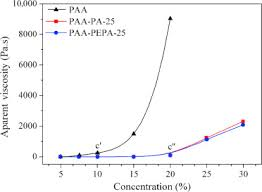The image above represents relative apparent viscosity (for concentrated suspension).

To compute for relative apparent viscosity (for concentrated suspension), three essential parameters are needed and these parameters are Intrinsic Viscosity (ηi), Solid Landing (φ) and Maximum Solid Landing (φm).

The formula for calculating relative apparent viscosity (for concentrated suspension):

ηra = (1 – φ / φm)-(ηim

Where:

ηra = Relative Apparent Viscosity
ηi = Intrinsic Viscosity
φ = Solid Landing
φm = Maximum Solid Landing

Let’s solve an example;
Find the relative apparent viscosity when the intrinsic viscosity is 20, the solid landing is 32 and the maximum solid landing is 12.

This implies that;

ηi = Intrinsic Viscosity = 20
φ = Solid Landing = 32
φm = Maximum Solid Landing = 12

ηra = (1 – φ / φm)-(ηim
ηra = (1 – 32 / 12)-(20) x 12
ηra = (1 – 2.66)-240
ηra = (-1.66)-240
ηra = 5.705e-54

Therefore, the relative apparent viscosity (for concentrated suspension) is 5.705e-54.

Nickzom Calculator – The Calculator Encyclopedia is capable of calculating the relative apparent viscosity (for concentrated suspension).

To get the answer and workings of the relative apparent viscosity (for concentrated suspension) using the Nickzom Calculator – The Calculator Encyclopedia. First, you need to obtain the app.

You can get this app via any of these means:

To get access to the professional version via web, you need to register and subscribe for NGN 2,000 per annum to have utter access to all functionalities.
You can also try the demo version via https://www.nickzom.org/calculator

Apple (Paid) – https://itunes.apple.com/us/app/nickzom-calculator/id1331162702?mt=8
Once, you have obtained the calculator encyclopedia app, proceed to the Calculator Map, then click on Materials and Metallurgical under Engineering.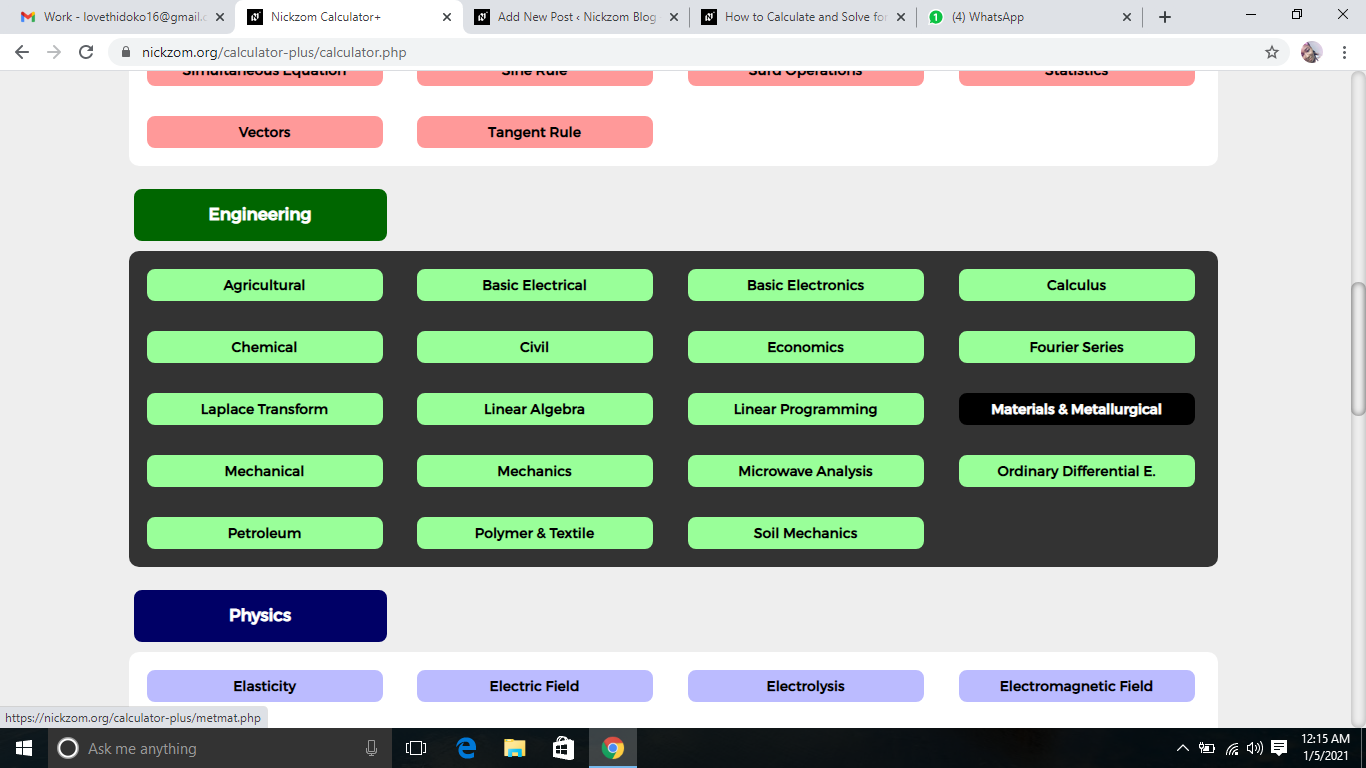Now, Click on Rheology under Materials and Metallurgical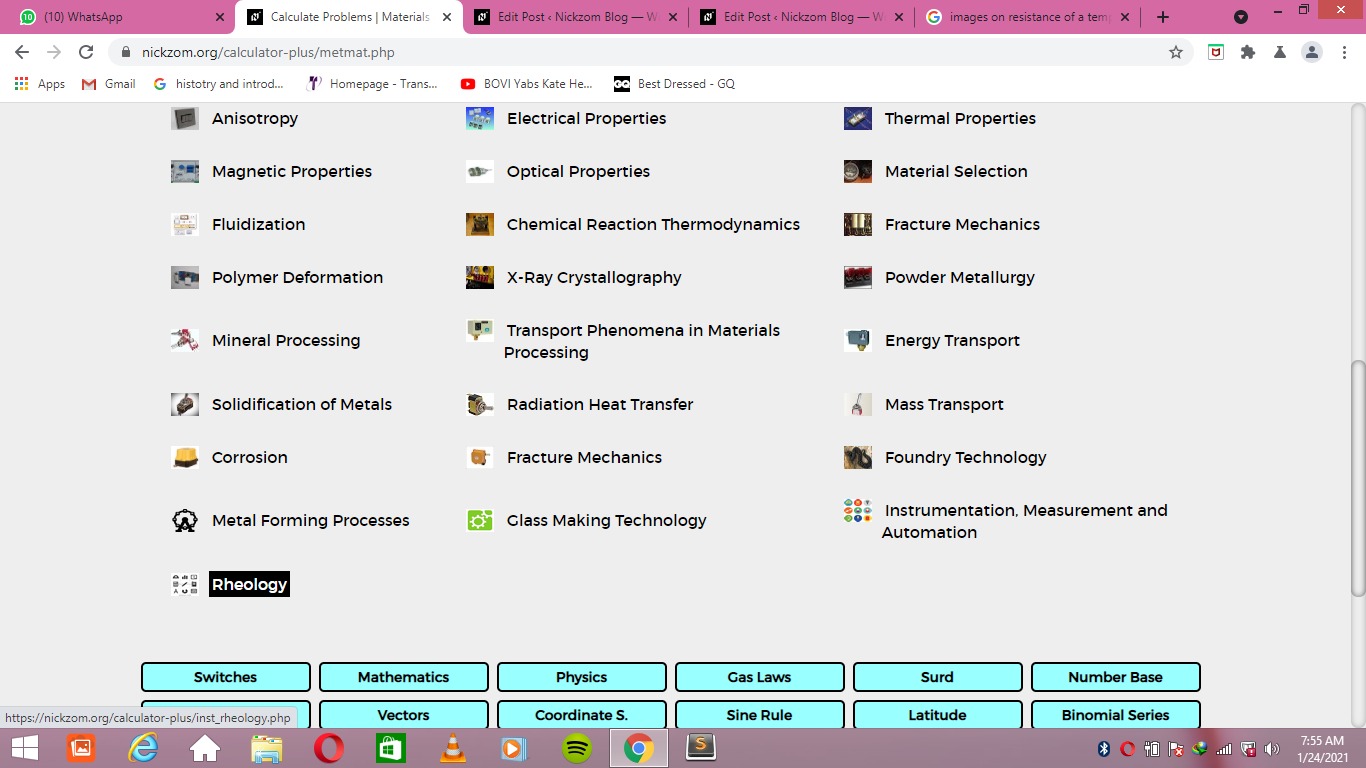Now, Click on Relative Apparent Viscosity (for concentrated suspension) under Rheology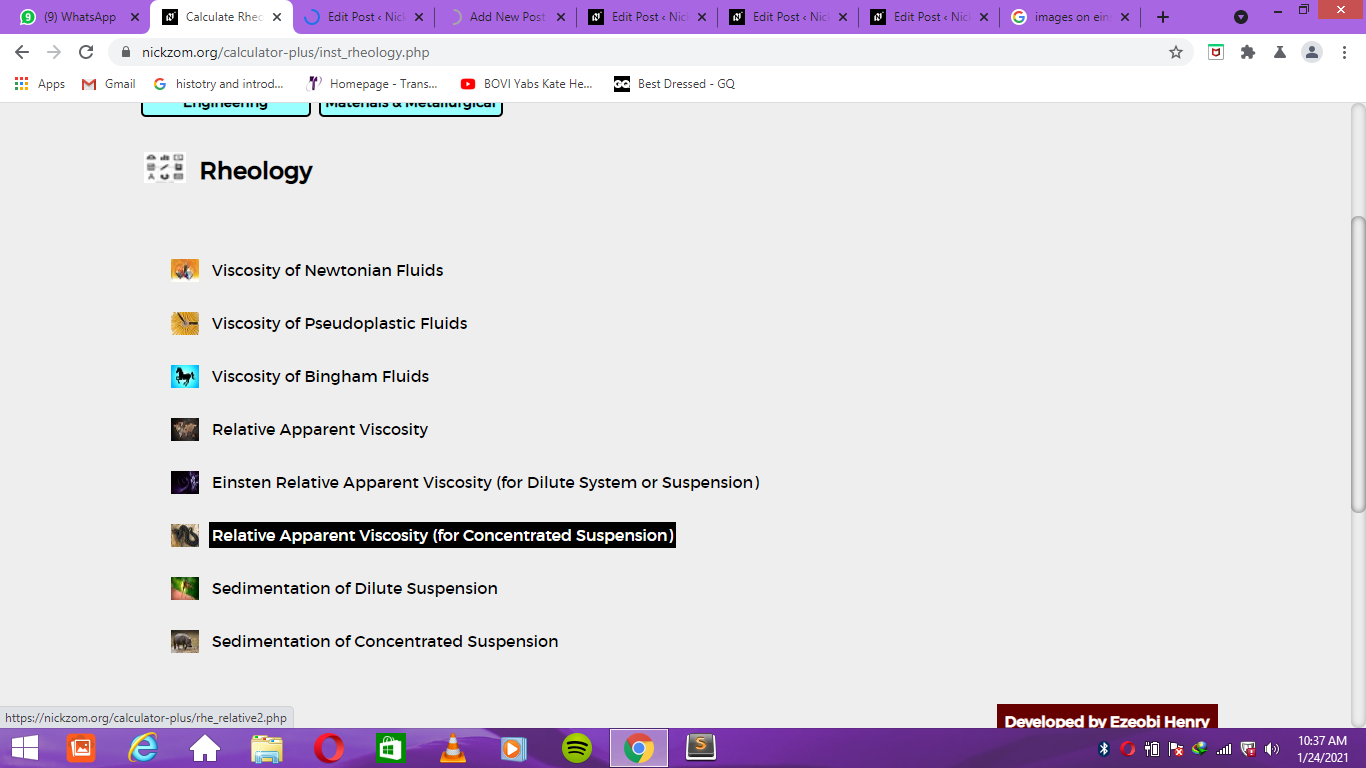The screenshot below displays the page or activity to enter your values, to get the answer for the relative apparent viscosity (for concentrated suspension) according to the respective parameters which is the Intrinsic Viscosity (ηi), Solid Landing (φ) and Maximum Solid Landing (φm).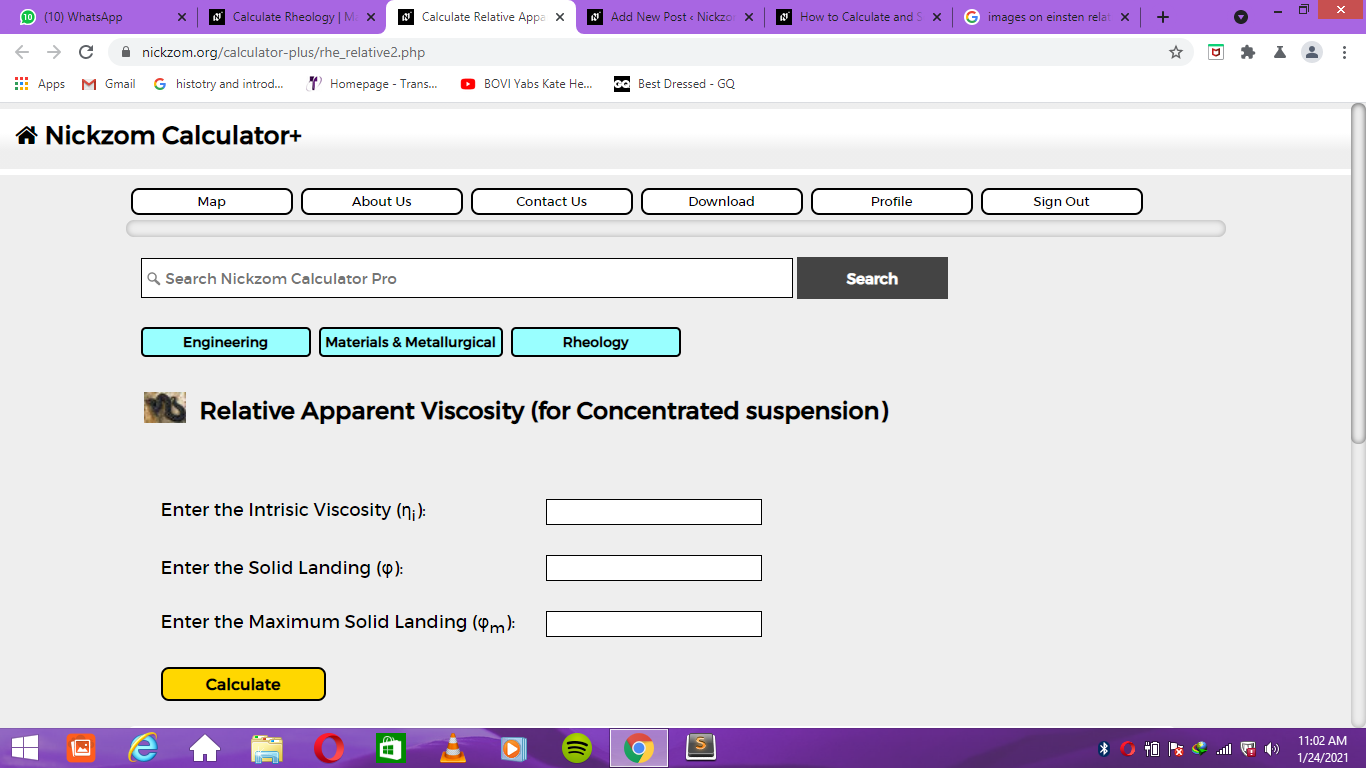Now, enter the values appropriately and accordingly for the parameters as required by the Intrinsic Viscosity (ηi) is 20, Solid Landing (φ) is 32 and Maximum Solid Landing (φm) is 12.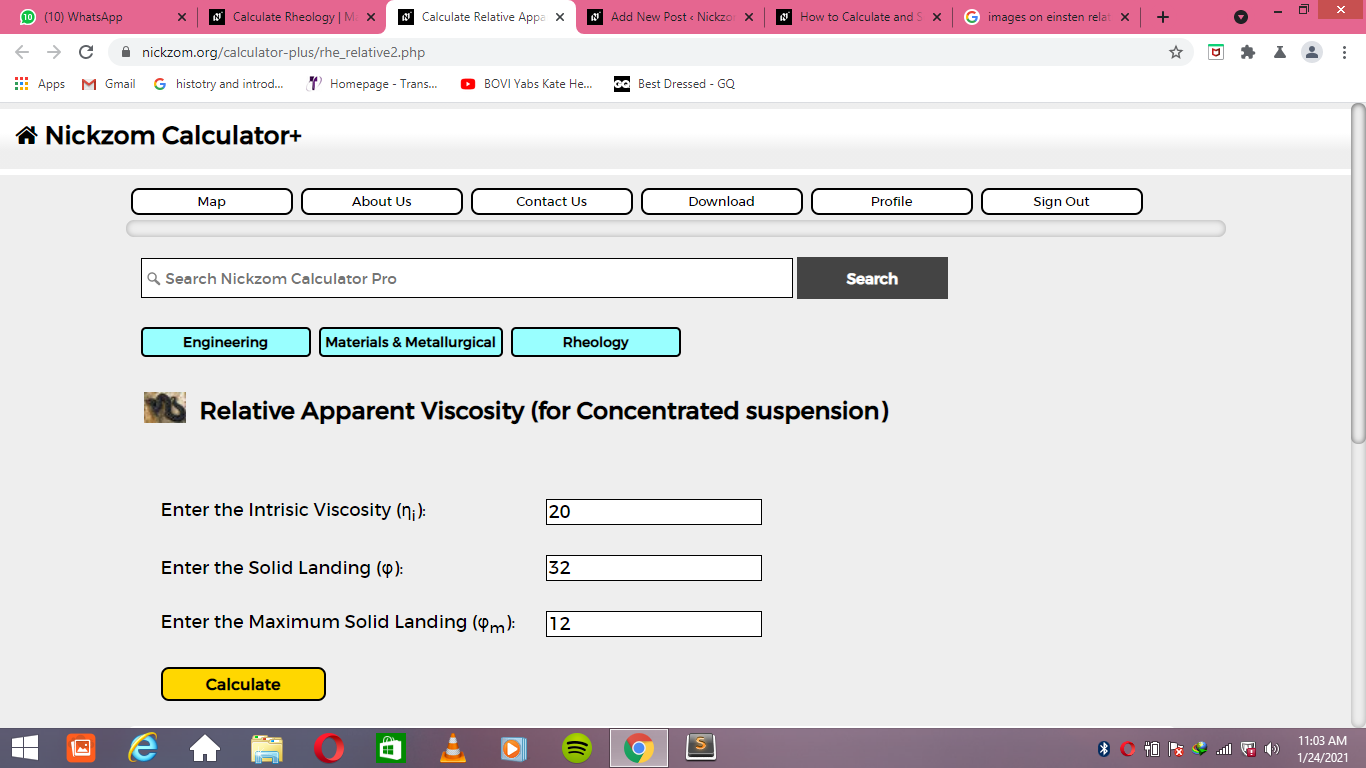Finally, Click on Calculate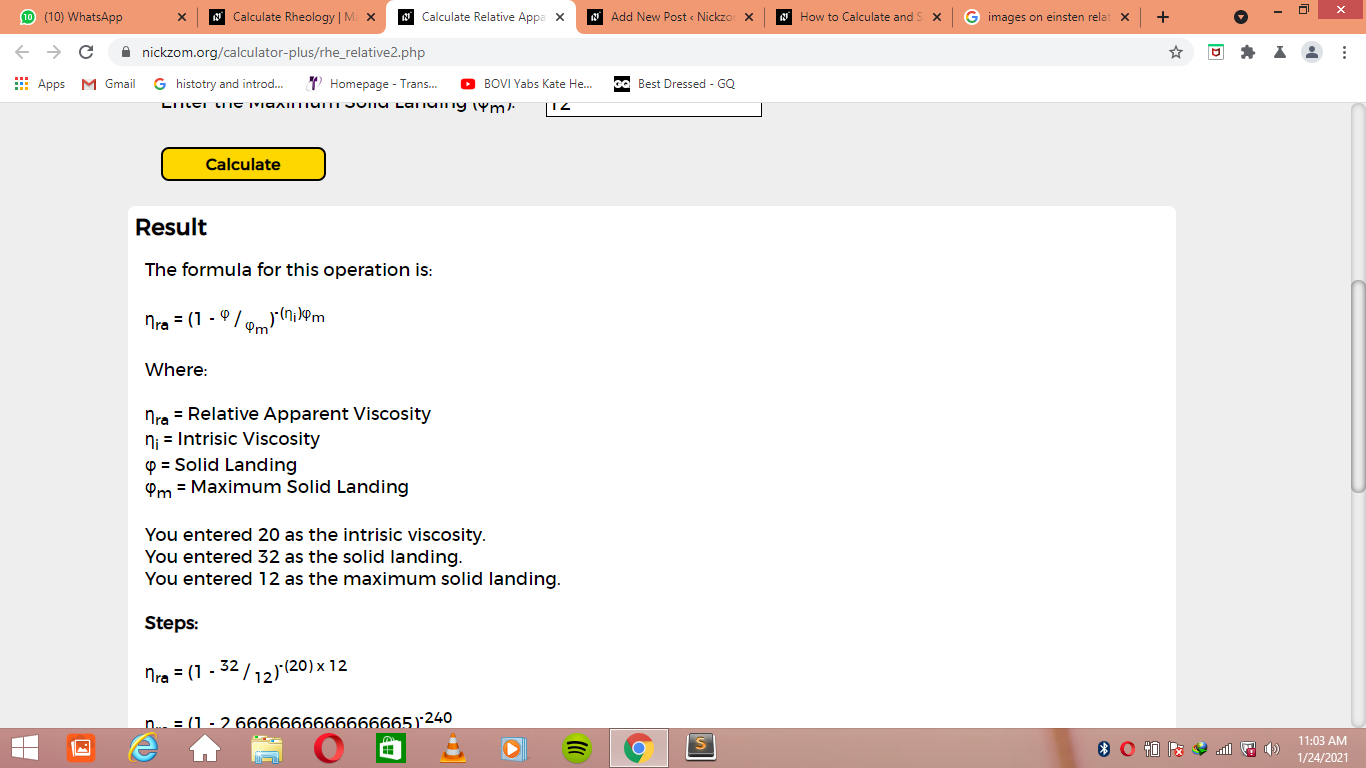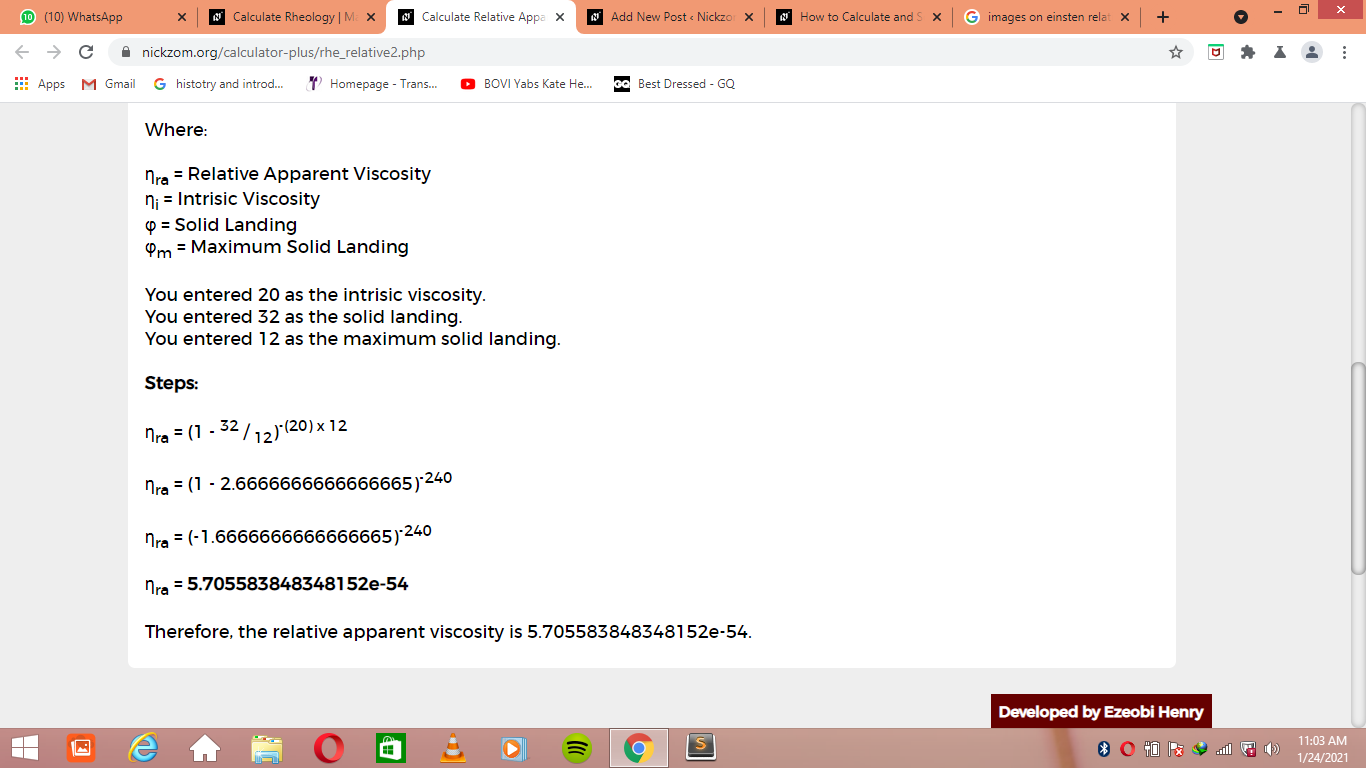As you can see from the screenshot above, Nickzom Calculator– The Calculator Encyclopedia solves for the relative apparent viscosity (for concentrated suspension) and presents the formula, workings and steps too.